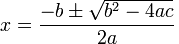Posted in Science & Nature

Anyone who has studied mathematics to some degree will know about algebraic equations. An algebraic equation is an equation that can be solved to find the unknown value of x. A quadratic equation is an algebraic equation with , or in other words has two valid solutions to x. Generally speaking, a quadratic equation can be expressed in the following fashion: ax² + bx + c = 0. a, b and c are constants and the equation can be solved to find x. A quadratic equation is definitely more complicated to solve compared to a linear equation and it can be solved using various means and applications such as factorisation. As these methods are learnt in school and this Encyclopaedia is technically not a mathematics textbook, such methods will not be delved into.

If you have not learnt it already, there is a shortcut method to solving quadratic equations: the quadratic formula. This formula can easily find x if you simply substitute in the values for a, b and c. Of course this formula only works if the solutions are real numbers. The quadratic formula is as follows:As you can see, because of the ± sign, the formula can be used to find both solutions to a quadratic equation. Even without factorising, it can find the answer as long as you substitute numbers into it on a calculator, making maths class very easy. However, as mentioned above the Encyclopaedia of Absolute and Relative Knowledge is not a mathematics textbook and one should instead learn properly from their teacher, not using the formula until they have been taught it properly.Posted in Science & Nature

## Fermat’s Last Theorem

In the 17th century, a lawyer called Pierre de Fermat conjectured many theorems while reading a mathematics textbook called Arithmetica, written by an ancient Greek mathematician called Diophantus. He wrote his theorems on the margins of the books. After his death, a version of the Arithmetica with Fermat’s theorems was published and many mathematicians checked over Fermat’s proofs. However, there was one theorem that could not be solved. Fermat wrote on the theorem: “I found an amazing proof but it is too large to fit in this margin”.

Fermat’s last theorem is as follows:

No three positive integers x, y, and z can satisfy the equation
xⁿ + yⁿ = zⁿ for any integer value of n greater than two.

For example, x² + y² = z² can be solved using Pythaogorean triplets (e.g. 3, 4, 5) but there are no values for x, y and z that solves x³ + y³ = z³. This theorem remained unsolved for 357 years until Andrew Wiles finally found the proof in 1995.

There are many stories surrounding Fermat’s last theorem, but by far the most interesting is related to suicide. In 1908, a German mathematician called Paul Wolfskehl decided to kill himself after being cold-heartedly rejected by the woman he loved so much. He decided to shoot himself at midnight and in the remaining time started reading some mathematics texts until he found a flaw in Kummer’s theory, which disproved Cauchy and Lamé’s solution (the leading solution at the time. After Kummer’s essay, most mathematicians of the time gave up on Fermat’s last theorem). After researching Kummer’s essay, Wolfskehl found that it was far past midnight and he felt great pride in reinforcing Kummer’s solution. His depression was gone and through mathematics he found new meaning in his life. Wolfskehl, who believed that the theorem saved his life, made a resolution to donate his wealth to whoever solved Fermat’s last theorem, putting up 100,000 marks as a prize. This prize was claimed by Wiles in 1996 (then worth \$50,000).